# Equations in PowerPoint

PowerPoint 365
To create an effective presentation that contains equations, you need to use a special mathematical style. This style involves using special characters and syntax, a special type of line breaks, delimiters, and requirements for superscripts and subscripts.

PowerPoint offers the same Equation Editor tool as Microsoft Word and Excel for creating and formatting the beautifully designed equations you need: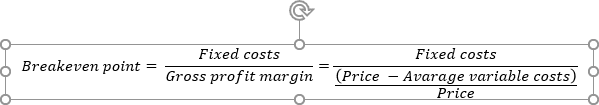or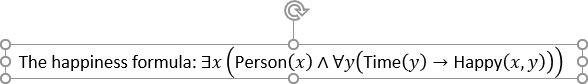## Insert an equation in the PowerPoint slide

Certain equations are pre-defined because they are used frequently in algebra and trigonometry, such as the Pythagorean Theorem and the Quadratic Formula. The Equation Editor offers to use pre-defined equations instead of re-creating them.

To insert the equation into the slide, do the following:

1.   Do one of the following:

• Select the placeholder or position the cursor in the text to insert the equation at the specified place.
• Unselect objects on a slide to create a new object for an equation. Thus, PowerPoint inserts a new text box in the middle of the slide.

2.   On the Insert tab, in the Symbols group, click the Equation button, and choose one of the equations from the gallery: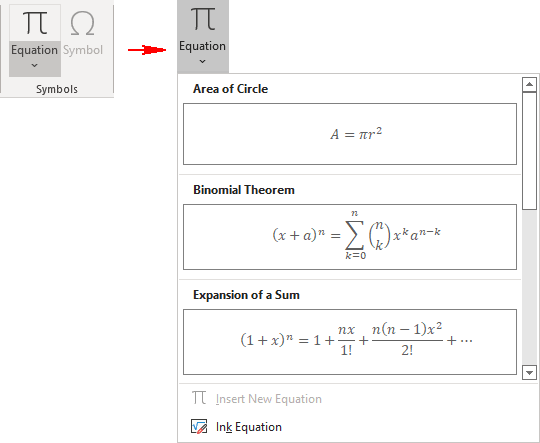The available predefined equations include:

• Area of Circle,
• Binomial Theorem,
• Expansion of a Sum,
• Fourier Series,
• Pythagorean Theorem,
• Taylor Expansion,
• Trig Identity 1,
• Trig Identity 2.

The equation is inserted into the existing text block or within a new text box on a slide: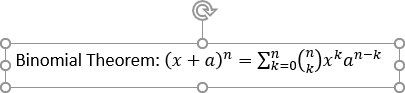To add an equation in the placeholder or into an object as a regular text, do the following:

1.   Position a cursor where you want to add a new equation.

2.   Do one of the following:

• On the Insert tab, in the Symbols group, click the Equation button: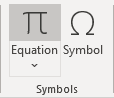• Click Alt+=.

PowerPoint adds text Type equation here right after where the cursor was: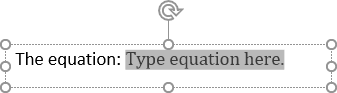## Create a new equation

To create a new text box with a new equation, do the following:

1.   Make sure that no objects are selected on a slide.

2.   On the Insert tab, in the Symbols group, click the Equation button (click its face, not its down arrow):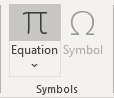PowerPoint creates a new text box in the middle of the slide with the Type equation here placeholder for a new equation:## Working with equations

To create a simple equation, just start typing it in the Type equation here placeholder. You can use any number, letter, or symbol from the keyboard.

To insert any of a wide variety of the math symbols, select them from the Symbols group on the Equation tab (it appears when you position the cursor into an equation placeholder):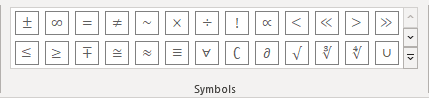Click the More button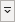to choose a different set of symbols if necessary: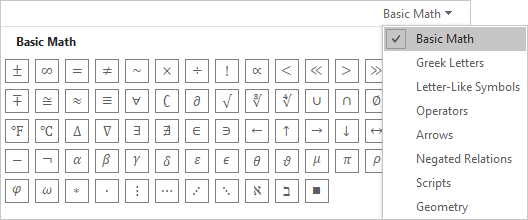For example, the Geometry set: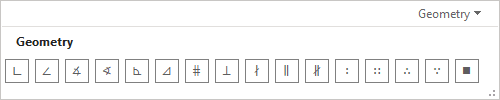The Equation tab offers to insert templates for various structures. Structures are symbols or combinations of text placeholder boxes that help you create mathematical equations that could not be easily expressed on a single line of text: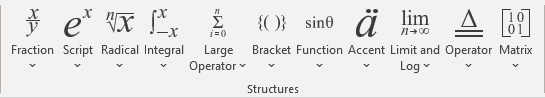When creating an equation, a structure is added, which is then filled with other structures, text, or symbols. According to Microsoft, there is no limit to the complexity of equations.

See more about equations in Word.

## Professional and Linear layout

The default equation layout type is Professional, which shows structures scattered across multiple lines where appropriate. A professional layout makes math formulas easy to read and understand.

Some users prefer to insert math and other symbols using shortcuts. To easily work with shortcuts and for another purpose, you can switch an equation to the Linear layout.

To switch between the Professional and Linear layouts of the equation, click the appropriate button on the Equation tab in the Tools group: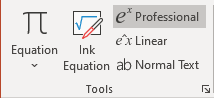and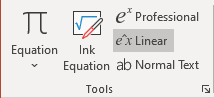The Professional format displays the equation on multiple line levels:Where the Linear form displays it on only one line: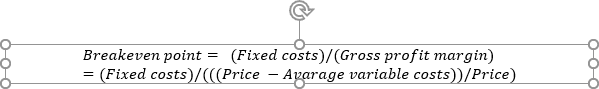## Formatting an equation

Even though equations look like specially formatted text, due to their nature, there are some differences in how equations and regular text are formatted:

• Font: The Cambria Math font is used for equations by default.

For regular text, you can easily change the font.

For equations, font changes will not affect if the font you choose does not support math symbols.

You can change the font only for symbols you marked as normal text. To do so, select a symbol or several symbols you want, then on the Equation tab, in the Tools group, click the Normal Text button: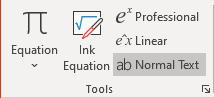So, you can change the font for all symbols for which the Normal Text button is highlighted.

Note: When you change the font for all text on the slide, it will be changed for all symbols in an equation that are marked as normal text as well: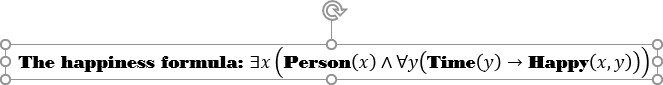• Size: By default, the baseline font for an equation is 18 pt. To maintain the display aspect ratio of an equation by changing the font size, you must change the font size for the entire equation, not for specific characters.
• Italic: Letters in an equation are italicized by default, as are some symbols. It's usually best to leave them at the default because people expect to see these items italicized, and the italics help them understand the equation.
• Underlining: Underlining cannot be applied to individual characters; it can only be applied to the entire equation.